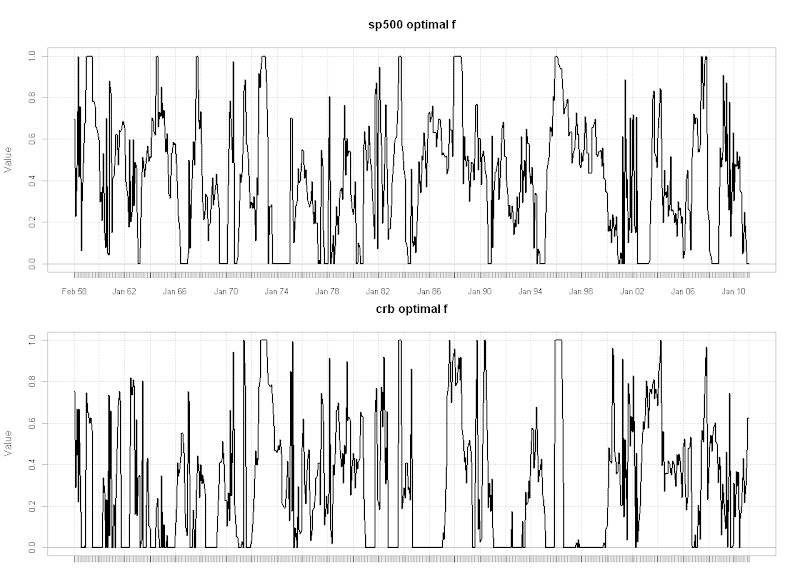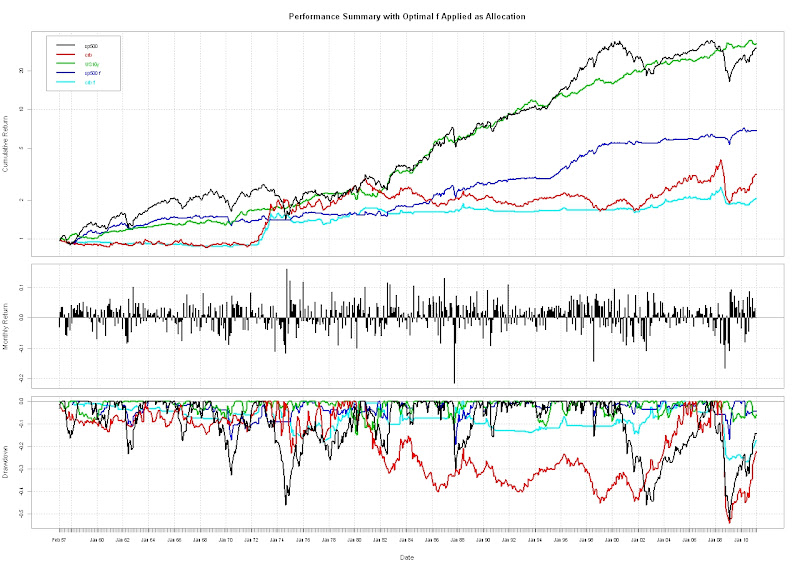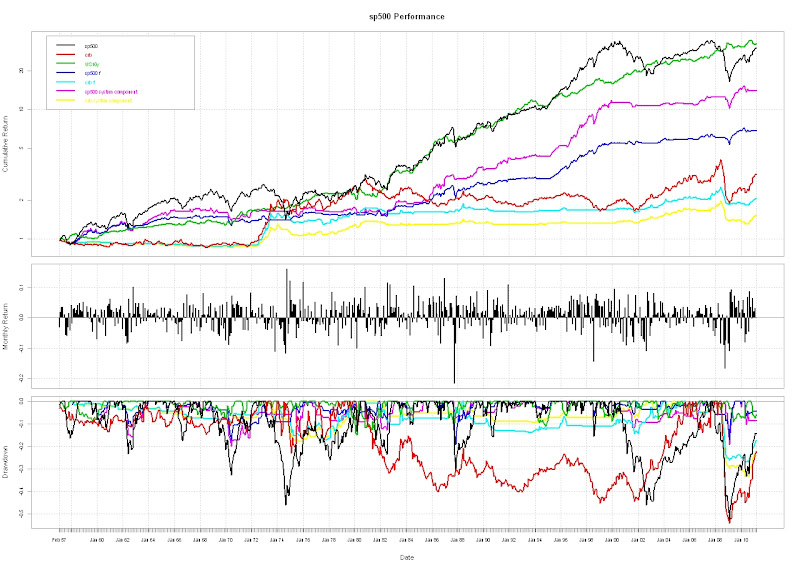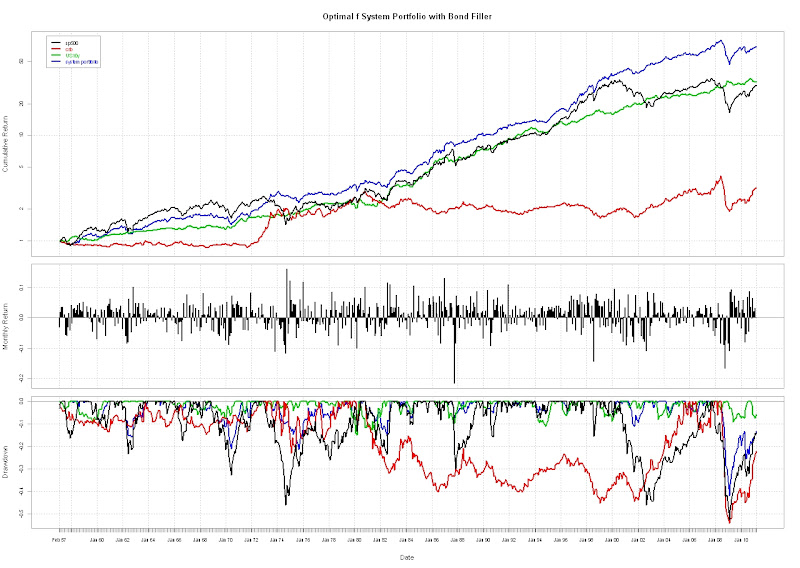Want to share your content on R-bloggers? click here if you have a blog, or here if you don't.

After the slightly unconventional use of LSPM presented in Slightly Different Use of Ralph Vince’s Leverage Space Trading Model, I thought I should follow up with something that more closely resembles my interpretation of Ralph Vince’s book.

LSPM seems to work well for portfolio allocation problems.  In this tactical allocation system, I will use optimal f derived in R with the LSPM package to build a portfolio with SP500, US10y, and the CRB.  If we use the optimal f as our allocation to SP500 and CRB, then the results look like this.From TimelyPortfolioFrom TimelyPortfolio

In general, my biggest problem is applying my systems to an entire portfolio.  The components are easy, but the blending is troublesome.  If I apply a basic method of monthly rebalancing of the SP500 and CRB, I get something like this (not using any leverage).From TimelyPortfolio

SP500 component improves, but because of my 50% limit on the more mean reverting CRB, the CRB component underperforms the straight optimal f allocation.From TimelyPortfolio

Since most clients don’t like cash, we can fill the portfolio with bonds when there is room left over after the CRB and SP500 allocation.  The total package look like this.From TimelyPortfolio

As always, I would like my posts to stimulate discussion and thought.  The drawdown here is much more severe than I would like.  Please let me know how you would improve this system.

R code:

#for original walkforward/optimize code and http://www.fosstrading.com
#for other techniques

require(PerformanceAnalytics)
require(quantmod)
require(RQuantLib)

#get bond returns to avoid proprietary data problems
#see previous timelyportfolio blogposts for explanation
#probably need to make this a function since I will be using so much
getSymbols(“GS10″,src=”FRED”) #load US Treasury 10y from Fed Fred

GS10pricereturn<-GS10  #set this up to hold price returns

GS10pricereturn[1,1]<-0
colnames(GS10pricereturn)<-“PriceReturn”
#I know I need to vectorize this but not qualified enough yet
#Please feel free to comment to show me how to do this
for (i in 1:(NROW(GS10)-1)) {
GS10pricereturn[i+1,1]<-FixedRateBondPriceByYield(yield=GS10[i+1,1]/100,issueDate=Sys.Date(),
rates=GS10[i,1]/100,period=2)/100-1
}

#interest return will be yield/12 for one month
GS10interestreturn<-lag(GS10,k=1)/12/100
colnames(GS10interestreturn)<-“Interest Return”

#total return will be the price return + interest return
GS10totalreturn<-GS10pricereturn+GS10interestreturn
colnames(GS10totalreturn)<-“Bond Total Return”

#get sp500 returns from FRED

#unfortunately cannot get substitute for proprietary CRB data
#get data series from csv file

#do a little manipulation to get the data lined up on monthly basis
GS10totalreturn<-to.monthly(GS10totalreturn)[,4]
SP500<-to.monthly(SP500)[,4]
#get monthly format to yyyy-mm-dd with the first day of the month
index(SP500)<-as.Date(index(SP500))
#my CRB data is end of month; could change but more fun to do in R
CRB<-to.monthly(CRB)[,4]
index(CRB)<-as.Date(index(CRB))

#now lets merge to get asset class returns
assetROC<-na.omit(merge(ROC(SP500,type=”discrete”),CRB,GS10totalreturn))

# Set Walk-Forward parameters (number of periods)
optim<-12 #1 year = 12 monthly returns
wf<-1 #walk forward 1 monthly returns

numsys<-2
# Calculate number of WF cycles
numCycles = floor((nrow(assetROC)-optim)/wf)
# Define JPT function
# this is now part of LSPM package, but fails when no negative returns
# so I still include this where I can force a negative return
jointProbTable <- function(x, n=3, FUN=median, …) {
if(!require(LSPM,quietly=TRUE)) stop(warnings())

# handle case with no negative returns
for (sys in 1:numsys) {
if (min(x[,sys])> -1) x[,sys][which.min(x[,sys])]<- -0.03
}
# Function to bin data
quantize <- function(x, n, FUN=median, …) {
if(is.character(FUN)) FUN <- get(FUN)
bins <- cut(x, n, labels=FALSE)
res <- sapply(1:NROW(x), function(i) FUN(x[bins==bins[i]], …))
}
# Allow for different values of ‘n’ for each system in ‘x’
if(NROW(n)==1) {
n <- rep(n,NCOL(x))
} else
if(NROW(n)!=NCOL(x)) stop(“invalid ‘n'”)
# Bin data in ‘x’
qd <- sapply(1:NCOL(x), function(i) quantize(x[,i],n=n[i],FUN=FUN,…))
# Aggregate probabilities
probs <- rep(1/NROW(x),NROW(x))
res <- aggregate(probs, by=lapply(1:NCOL(qd), function(i) qd[,i]), sum)
# Clean up output, return lsp object
colnames(res) <- colnames(x)
res <- lsp(res[,1:NCOL(x)],res[,NCOL(res)])
return(res)
}

for (i in 0:(numCycles-1)) {
# Define cycle boundaries
start<-1+(i*wf)
end<-optim+(i*wf)
# Get returns for optimization cycle and create the JPT
# specify number of bins; does not seem to drastically affect results
numbins<-6
jpt <- jointProbTable(assetROC[start:end,1:numsys],n=rep(numbins,numsys))
outcomes<-jpt[]
probs<-jpt[]
port<-lsp(outcomes,probs)
# DEoptim parameters (see ?DEoptim)
np=numsys*10       # 10 * number of mktsys
imax=1000       #maximum number of iterations
crossover=0.6       #probability of crossover
NR <- NROW(port\$f)
DEctrl <- list(NP=np, itermax=imax, CR=crossover, trace=TRUE)
# Optimize f
res <- optimalf(port, control=DEctrl)
# use upper to restrict to a level that you might feel comfortable
#res <- optimalf(port, control=DEctrl, lower=rep(0,13), upper=rep(0.2,13))

# these are other possibilities but I gave up after 24 hours
#res<-maxProbProfit(port, 1e-6, 6, probDrawdown, 0.1, DD=0.2, control=DEctrl)
#res<-optimalf(port,probDrawdown,0.1,DD=0.2,horizon=6,control=DEctrl)

# Save leverage amounts as optimal f
# Examples in the book Ralph Vince Leverage Space Trading Model
# all in dollar terms which confuses me
# until I resolve I changed lev line to show optimal f output
lev<-res\$f[1:numsys]
#lev<-c(res\$f/(-jpt\$maxLoss/10),res\$f/(-jpt\$maxLoss/10))
levmat<-c(rep(1,wf)) %o% lev #so that we can multiply with the wfassetROC
# Get the returns for the next Walk-Forward period
wfassetROC <- assetROC[(end+1):(end+wf),1:numsys]
wflevassetROC <- wfassetROC*levmat #apply leverage to the returns
if (i==0) fullassetROC<-wflevassetROC else fullassetROC<-rbind(fullassetROC,wflevassetROC)
if (i==0) levered<-levmat else levered<-rbind(levered,levmat)
}

#not super familiar with xts, but this add dates to levered
levered<-xts(levered,order.by=index(fullassetROC) )
colnames(levered)<-c(“sp500 optimal f”,”crb optimal f”)
chart.TimeSeries(levered, legend.loc=”topleft”, cex.legend=0.6)

#review the optimal f values
#I had to fill the window to my screen to avoid a error from R on margins
par(mfrow=c(numsys,1))
for (i in 1:numsys) {
chart.TimeSeries(levered[,i],xlab=NULL)
}

#charts.PerformanceSummary(fullassetROC, ylog=TRUE, main=”Performance Summary with Optimal f Applied as Allocation”)

assetROCAnalyze<-merge(assetROC,fullassetROC)
colnames(assetROCAnalyze)<-c(“sp500″,”crb”,”US10y”,”sp500 f”,”crb f”)
charts.PerformanceSummary(assetROCAnalyze,ylog=TRUE,main=”Performance Summary with Optimal f Applied as Allocation”)

#build a portfolio with sp500 and crb
#allow up to 50% allocation in CRB
#allow up to 100% allocation in SP500 but portfolio constrained to 1 leverage
colnames(assetROCadjust)<-c(“sp500″,”crb”,”US10y”,”sp500 f”,”crb f”,”sp500 system component”,”crb system component”)

#review the allocations versus optimal f
#I had to fill the window to my screen to avoid a error from R on margins
par(mfrow=c(numsys,1))
for (i in 1:numsys) {
}

#add bonds when out of sp500 or crb